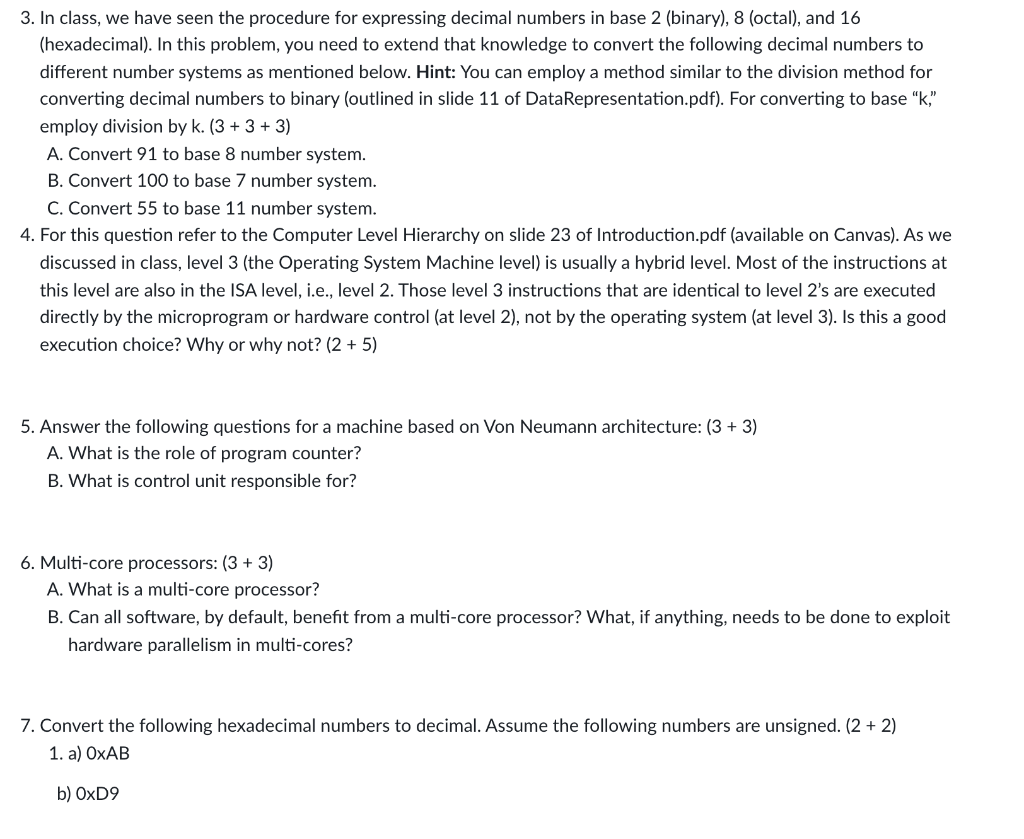Rating : ⭐⭐⭐⭐⭐
Price : \$10.99
Language:EN
Pages: 5
Words: 794

# Number Conversions and Architecture Assignment Answers3. In class, we have seen the procedure for expressing decimal numbers in base 2 (binary), 8 (octal), and 16 (hexadecimal). In this problem, you need to extend that knowledge to convert the following decimal numbers to different number systems as mentioned below. Hint: You can employ a method similar to the division method for converting decimal numbers to binary (outlined in slide 11 of DataRepresentation.pdf). For converting to base “K," employ division by k. (3+3+3) A. Convert 91 to base 8 number system. B. Convert 100 to base 7 number system. C. Convert 55 to base 11 number system. 4. For this question refer to the Computer Level Hierarchy on slide 23 of Introduction.pdf (available on Canvas). As we discussed in class, level 3 (the Operating System Machine level) is usually a hybrid level. Most of the instructions at this level are also in the ISA level, i.e., level 2. Those level 3 instructions that are identical to level 2's are executed directly by the microprogram or hardware control (at level 2), not by the operating system (at level 3). Is this a good execution choice? Why or why not? (2 + 5) 5. Answer the following questions for a machine based on Von Neumann architecture: (3+3) A. What is the role of program counter? B. What is control unit responsible for? 6. Multi-core processors: (3 + 3) A. What is a multi-core processor? B. Can all software, by default, benefit from a multi-core processor? What, if anything, needs to be done to exploit hardware parallelism in multi-cores? 7. Convert the following hexadecimal numbers to decimal. Assume the following numbers are unsigned. (2 + 2) 1. a) OXAB b) OxD9

# Assignment Help Answers with Step-by-Step Explanation:

91 ÷ 8 = 11 remainder 3

11 ÷ 8 = 1 remainder 3

100 ÷ 7 = 14 remainder 2

14 ÷ 7 = 2 remainder 0

55 ÷ 11 = 5 remainder 0

5 ÷ 11 = 0 remainder 5

A. Role of program counter (PC):

The program counter is a register in the CPU that keeps track of the address of the next instruction to be executed. It points to the memory location where the next instruction is stored. The PC is crucial for the sequential execution of instructions in a program.

A multi-core processor is a CPU that contains two or more independent processing units (cores) on a single chip. Each core can execute instructions independently and in parallel, allowing for better multitasking and increased processing power.

B. Can all software benefit from a multi-core processor? What needs to be done to exploit hardware parallelism in multi-cores?

0xAB = (A * 16^1) + (B * 16^0)

= (10 * 16^1) + (11 * 16^0)

Similarly, for 0xD9:

0xD9 = (D * 16^1) + (9 * 16^0)

So, the decimal representations are:

a) 0xAB = 171

How It Works